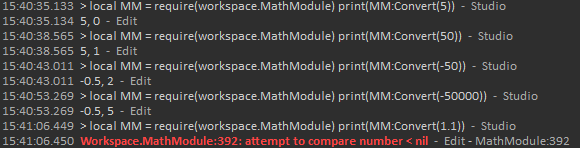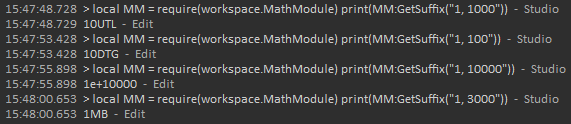# Alternatives to BigNum that store integers and decimals

First post I’ve made on devforum for support, I’m honestly just lost at this point and need someone who can hopefully offer a solution.
Basically the problem I’m facing is that currently, me and a few friends are working on an idle game (similar to cookie clicker) and we initially needed a way to store values with precision above 1.e+21 (Roblox’s number limit, or close to). We came across the BigNum library from RoStrap, and that worked well until we came into another issue, it just doesn’t support decimals.
Essentially, we use decimals to create a fair, balanced progression system, so when you buy an upgrade it follows a formula of n*1.5 (n being the price of the upgrade) on every purchase, however, BigNum is unable to support that, instead returning a pseudo math.floor on every calculation, with no way to even use decimals in calculations.

I tried creating BigNum floats by adding a .0 to the end of any integers, but that didn’t work either, instead, returning whole number integers after calculations, and at this point, I’m kinda lost.
Would be dope if someone could send me in the direction of a library (if it exists) that both supports numbers and math above Roblox’s number limit and support for decimal calculations.

1 Like

Represent it ℚ instead. Represent `1.5` as `3/2` and `{ x | x ∈ ℤ }` as `x/1`. You ought to know how to add and multiply rationals. To do with create a class with the numerator and denominator, both being an arbitrary precision integer data type.

All values with finite decimal expansion can be represented in ℚ.

BigNum only supports integers.

The reason its not storing decimals is because Integers don’t support decimals. You need to be using a Float instead which has a limit of 1 * 10^308, or 1 with 308 zeros, and supports decimals. Instead of using an IntValue for storing your numbers you need to use a NumberValue.

But if you want to go above 1 * 10^308, I’ve actually made a system for that. But it doesn’t actually store numbers, it stores strings. Basically it stores the first part of the number, and the amount of 0’s separately, meaning it doesn’t hit the limit because 1 * 10^1000(would be inf) is just “1, 1000”.

My system also has suffix support up until 1 * 10^3000, the last suffix being ‘MTG’. I could add more, but its a manual process and I don’t really need to go that high anyway.

It can go up to 1 * 10^(1 * 10^308), or 1 with 1 * 10^308 zeros, or 1 with 1 centillion zeros. It also can hit this lag free, I’ve made a live game using this system before and its practically lag free because the actual math being done is basically just like normal.

The reason for my systems limit here is because once we go above 1 * 10^308 zeros, we hit inf zeros, which is just like the normal roblox inf. But of course this limit is so incredibly high it’ll never get hit unless you intentionally hit it.

There are other limits with my system, like it still doesn’t support decimals, I’m sure decimal support could be added but I’ve lost interest in adding it.

Here you see a function called Convert which lets you convert regular numbers into MathNumbers, it can handle regular numbers and even negatives, but errors with a decimal.We also have GetSuffix, which would turn something like 1000 into 1k for displaying easily. Once you go above the suffixes in the module it just returns scientific notation.Here’s the file for it if you’d like, but if you’re trying to get above 21 zeros using NumberValues should probably be fine for you.
MathModule.rbxm (7.4 KB)

In respone to your first paragraph, I’m aware Integers do not support decimals, and had been storing my values in NumberValues. The problem I run into there is that storing whole numbers - decimals or not - above 1.e+21 would simply output the scientific notation when calling it, and would utilise an inaccurate number for calculations, only to just output scientific notation when called again.

The system you’ve provided to me throughout the rest is essentially just BigNum, the same library I’ve been using already, storing numbers in strings above the default roblox number limit, without the capability to handle decimal calculations.

Additionally, I already have a system implemented that converts numbers such as 1,000 and 1,000,000 into their respective suffixes. I appreciate the effort and system, but ultimately, doesn’t get me anywhere closer to finding a proper solution.

There is no reason a game should ever need numbers that big, stop complicating things for no reason.

But you can store the numbers as strings but then you would have to make your own addiction subtraction division and mutiplication functions, the only hard one would be division

On the contrary, I disagree. I have an idle game in the works and aim to store numbers over a certain point (1.e+21), so yes, I do need numbers that big.

I’m aware I can store numbers in strings, and I already have a library that handles addition, subtraction, multiplication and division (BigNum), however, when it comes to incrementing my upgrades, I require it to multiply by a decimal, as the lowest multiplication vector above 1 is 2, which is ridiculously absurd in comparison to my reference, Cookie Clicker.

I believe I made that intention clear from the start also, so if you’re just here to make pointless comments and offer no assistance whatsoever, then please refrain from making comments at all. Thank you.

here is a simplified version on the code below to help you understand what the complex version is doing

``````local number = 0
local thousands = 0
local millions = 0
local billions = 0

-- as long as you don't add very large numbers this should be accurate
number += value

local floor = math.floor(number/1000)
number -= floor * 1000
thousands += floor

local floor = math.floor(thousands/1000)
thousands -= floor * 1000
millions += floor

local floor = math.floor(millions/1000)
millions -= floor * 1000
billions += floor
end

-- this might not be accurate as we are compressing all variables into one
local function GetValue()
return number + thousands * 1000 + millions * 1000000 + billions * 1000000000
end

-- this might not be accurate as we are compressing variables into one
local function GetString()
if billions > 0 then
return billions + millions / 1000 .. "B"
elseif millions > 0 then
return millions + thousands / 1000 .. "M"
elseif thousands > 0 then
return thousands + number / 1000 .. "K"
else
end
end

local random = Random.new()
local value = 0
for i = 1, 5000 do
local r = random:NextNumber(10000000000, 1000000000000)
value += r
end

print(value)
print(GetValue())
print(GetString())
``````

and here is a more complex version

``````local abbreviations = {"", "K", "M", "B", "T", "Q"}
local numbers = {0, 0, 0, 0, 0, 0}

-- as long as you don't add very large numbers this should be accurate
numbers += value
for i = 2, #numbers do
local floor = math.floor(numbers[i-1] / 1000)
numbers[i-1] -= floor * 1000
numbers[i] += floor
end
end

-- this might not be accurate as we are compressing all variables into one
local function GetValue()
local value = 0
for i = 1, #numbers do
value += numbers[i] * 1000 ^ (i - 1)
end
return value
end

-- this might not be accurate as we are compressing all variables into one
local function GetAbbreviations()
for i = #numbers, 1, -1 do
if numbers[i] == 0 then continue end
local value = 0
local count = 0
for j = i, 1, -1 do
value += numbers[j] / (1000 ^ count)
count += 1
end
return value .. abbreviations[i]
end
end

-- this will be accurate
local function GetString()
for i = #numbers, 1, -1 do
if numbers[i] == 0 then continue end
local numberString = tostring(numbers[i])
for j = i-1, 1, -1 do
for k = 1, 3 - #tostring(math.floor(numbers[j])) do
numberString = numberString .. "0"
end
numberString = numberString .. numbers[j]
end
return numberString
end
end

local random = Random.new()
local value = 0
for i = 1, 1000000 do
local r = random:NextNumber(10000000000, 1000000000000)
value += r
end

print(value)
print(GetValue())
print(GetAbbreviations())
print(GetString())
print(table.unpack(numbers))
``````

these are some results i get

``````505395016751998460
505395016751973900
505.3950167519739Q
505395016751973917.3310546875
917.3310546875 973 751 16 395 505
-------------------------------
50420742629934980
50420742629935860
50.420742629935866Q
50420742629935858.1500854492188
858.1500854492188 935 629 742 420 50
-------------------------------
502723097982830.1
502723097982830.6
502.7230979828306T
502723097982830.623046875
830.623046875 982 97 723 502 0
-------------------------------
4363427958804.767
4363427958804.7676
4.363427958804768T
4363427958804.7675170898438
804.7675170898438 958 427 363 4 0
``````

Why not use BigNum, but store everything as 1000X the size?

For instance, if you have “50” gold, internally you store that as `BigNum.new(50 * 1000)`.

Then the smallest multiplier you have is “0.001”, or `BigNum.new(0.001 * 1000)`.

Only time you need to convert is when displaying the number to the user.

Wouldn’t be a ton of work to wrap that in a library yourself.

2 Likes

Exactly what i was trying to say by the there is no reason for such presision. There is no difference between 1.1 and 11 just scale you application by 10

While that should work in theory, it brings up the same error I always get with BigNum when attempting to do any kind of arithmetic involving whole numbers and decimals.In essence, it’s telling me that a decimal has a radix of 31, and a whole number has a radix of 32. A “solution” to this would be to multiply everything inside of a single “BigNum.new” statement, and while this works in some cases, in a few cases, it does not. Unfortunately those cases provide a problem to the project I’m working on.
In this scenario, the value of an upgrade went from 10 to 15 using this method seamlessly, but when attempting it again, the output was 0 where it should have been 22.5, and this is where the problem lies. It does not give me a chance to retain the .5 at the end of the string, and instead, just returns 0.In this scenario, “val.Value” is equal to 15.

So I appreciate the input, but yet again, does not work, as I knew from hours of excessive testing to find some kind of a solution. This is why I’m asking if anyone knows any library other than BigNum that I can use, rather than offering a new method of thinking that I’ve already tried.
Additionally, if it helps anyone else, I’m not attempting to store a currency, that is fine and already working, and I can store numbers well above integer limit, I’m more on about precise accuracy with decimals for upgrades, which increase by a set decimal each time, (as the lowest integer above 1 (which is 2) is far too absurd to actually expect people to play with) but when you get into the late game, the upgrade costs will far exceed the Roblox number limit.